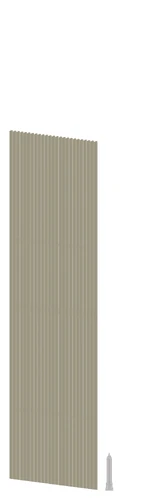11,052 PagesA sptdoocol dollars in 25 piles of 100 dollar bills, in comparison to the Empire State Building.

The sptdoocol or 17-noogol is equal to s(10,17) using strong array notation, or 1017 = 100,000,000,000,000,000.  The term was coined by Aarex Tiaokhiao.

This number is called a shankh in the indian counting system.

## As a banknote denomination

Only the Hungarian pengő had banknotes with this number in the denomination.

## Approximations

Notation Lower bound Upper bound
Scientific notation $$1\times10^{17}$$
Arrow notation $$10\uparrow17$$
Steinhaus-Moser Notation 14 15
Copy notation 9 10
Taro's multivariable Ackermann function A(3,53) A(3,54)
Pound-Star Notation #*(5,3,2,1)*4 #*(5,2,2)*6
BEAF {10,17}
Hyper-E notation E17
Bashicu matrix system (0)(0) (0)(0)
Hyperfactorial array notation 18! 19!
Fast-growing hierarchy $$f_2(50)$$ $$f_2(51)$$
Hardy hierarchy $$H_{\omega^2}(50)$$ $$H_{\omega^2}(51)$$
Slow-growing hierarchy $$g_{\omega^{\omega+7}}(10)$$

## Sources

Community content is available under CC-BY-SA unless otherwise noted.